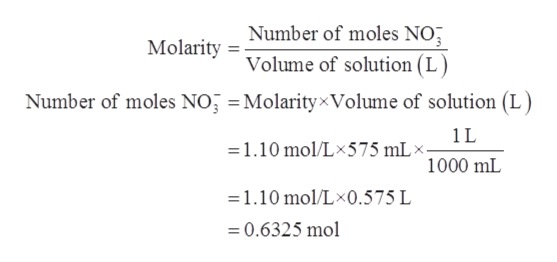# When 575 mL of a solution that is 1.10 M in NO3– reacts with excess Cr(OH)3 solid, how many moles of electrons are transferred during the reaction?

Question
7 views

When 575 mL of a solution that is 1.10 M in NO3 reacts with excess Cr(OH)3 solid, how many moles of electrons are transferred during the reaction?

check_circle

Step 1

Given information:

Volume of solution (V) = 575 mL

Concentration or molarity of solution (M) = 1.10 M

Step 2

Molarity is defined as the number of moles of solute dissolved in 1 liter of solution. It is represented by M. Its unit is mol/L.

The expression for molarity is given as follows:

Step 3

Thus, the number of moles of NO3- are cal...help_outlineImage TranscriptioncloseNumber of moles NO Volume of solution (L MolarityxVolume of solution (L Molarity Number of moles NO 1L =1.10 mol/Lx575 mL 1000 mL 1.10 mol/Lx0.575 L 0.6325 mol fullscreen

### Want to see the full answer?

See Solution

#### Want to see this answer and more?

Solutions are written by subject experts who are available 24/7. Questions are typically answered within 1 hour.*

See Solution
*Response times may vary by subject and question.
Tagged in

### General Chemistry